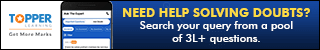Request a call back

# Class 10 SELINA Solutions Maths Chapter 11 - Geometric Progression

## Geometric Progression Exercise Ex. 11(A)

### Solution 1(i)### Solution 1(ii)### Solution 1(iii)### Solution 2### Solution 3### Solution 4### Solution 5### Solution 6### Solution 7### Solution 8### Solution 9### Solution 10### Solution 11### Solution 12

Given series: 2, - 6, 18, - 54 ……## Geometric Progression Exercise Ex. 11(B)

### Solution 1### Solution 2### Solution 3### Solution 4### Solution 5### Solution 6### Solution 7### Solution 8### Solution 9### Solution 10## Geometric Progression Exercise Ex. 11(C)

### Solution 1### Solution 2### Solution 3### Solution 4### Solution 5### Solution 6### Solution 7### Solution 8(i)### Solution 8(ii)### Solution 9## Geometric Progression Exercise Ex. 11(D)

### Solution 1(i)### Solution 1(iii)### Solution 1(iv)### Solution 1(v)### Solution 1(vi)### Solution 2### Solution 3### Solution 4### Solution 5### Solution 6### Solution 7### Solution 8### Solution 9### Solution 10### Solution 11### Solution 12(i)### Solution 12(ii)### Solution 12(iii)### Solution 13### Solution 14### Solution 15

First term (a) =### Solution 16

First term (a) = 125### Solution 17Thus, the given sequence is a G.P. with### Solution 18### Solution 19

Let the five terms of the given G.P. beGiven, sum of first two terms = -4And, 5th term = 4(3rd term)

ar2 = 4(a)

r2 = 4

r = ±2

When r = +2,When r = -2,### Solution 1(ii)